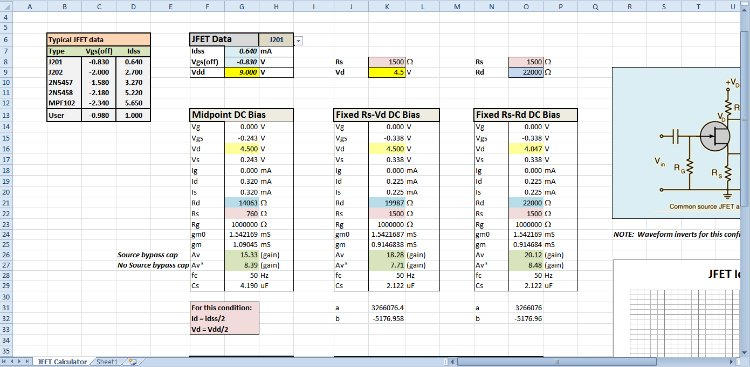JFET common source gain stage calculator ver 2.1ClassicListThreaded2 messagesThis post was updated on . Here is the latest version of my JFET calculator spreadsheet (Excel).  There are some minor tweaks, including a drop-down list so you can select a specific JFET from the data. The two main calculations are the given source resistor (Rs) and drain bias voltage (Vd) calculation and the given Rs and drain resistor (Rd) calculation.  You supply the JFET data and supply voltage (9V).  The calculator will do the rest.  Note that the gains are calculated both with and without a source bypass capacitor.  There's even a Fetzer bias calculation! The main use of this tool is to determine the appropriate drain resistor for a given source resistor and drain bias voltage.  Knowing this you potentially build effects like the Dr. Boogie or the Plexidrive without the need to trimmers (as long you measure the JFET data for YOUR JFETs and supply it to the spreadsheet). Have fun!JFET Calculator (Excel)Administrator Great as always frank.